#### The complete guide to Seaborn for your beautiful plotting needs

Seaborn is a library for creating beautiful visualizations in Python. It is built on top of matplotlib and provides a high-level API for drawing pretty statistical graphs. At first, using the library can seem daunting. However, with consistent practice, you will get the hang of it. To this end, I have created a comprehensive Seaborn cheat sheet that you can consistently refer to as you get familiar with the library.Photo by Nicholas Cappello on Unsplash

### Table of Content:

1. Overview
2. Data Preparation
3. Figure Aesthetics
4. Plotting: Categorical
5. Plotting: Regression
6. Plotting: Distribution & Matrix
7. Plot Customization
8. Show and Save Plot
9. Bonus

### Overview

This will be the basic step to creating plots with Seaborn.

`>>> import matplotlib.pyplot as plt>>> import seaborn as sns`
`# DATA PREPARATION>>> tips = sns.load_dataset("tips")`
`# FIGURE AESTHETICS>>> sns.set_style("whitegrid")`
`# PLOTTING>>> g = sns.lmplot(x="tip", y="total_bill", data=tips, aspect=2)`
`# CUSTOMIZE PLOT>>> g = (g.set_axis_labels("Tip", "Total bill (USD)"))`
`# SHOW and SAVE PLOT>>> plt.savefig('foo.png')>>> plt.show(g)`

### Data Preparation

Built-in datasets

`>>> titanic = sns.load_dataset("titanic")>>> iris = sns.load_dataset("iris")`

Your Own Dataset

`# You can build your dataset using Pandas DataFrame or Numpy array objects`

### Figure Aesthetics

Create Figure and Subplots

`# Creating one figure and one subplot>>> f, ax = plt.subplots()`
`# With a pre-defined figure size>>> f, ax = plt.subplots(figsize=(6,6))`

Reset Style

`>>> sns.set()`

Set Style

There are five preset seaborn themes: darkgrid, whitegrid, dark, white, and ticks

`>>> sns.set_style("darkgrid")>>> sns.set_style("whitegrid")>>> sns.set_style("dark")>>> sns.set_style("white")>>> sns.set_style("ticks")`

Set Color

Full list: Color Palette

`>>> sns.set_palette("husl", 3)`

### Plotting: Categorical

Scatterplot: Stripplot (one categorical variable)

`>>> sns.stripplot(x="species", y="petal_length", data=iris)`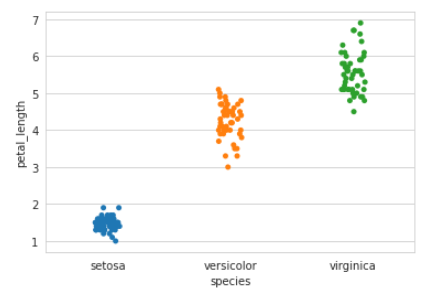Strip Plot Graph Example

Scatterplot: Swarmplot (non-overlapping points)

`>>> sns.swarmplot(x="species", y="petal_length", data=iris)`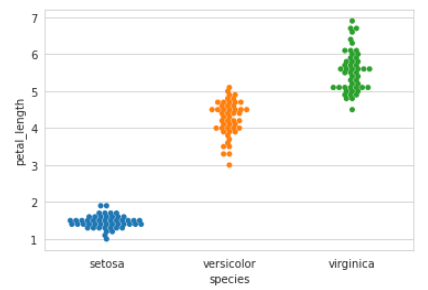Swarmplot Graph Example

Bar Chart

`>>> sns.barplot(x="sex", y="survived", data=titanic)`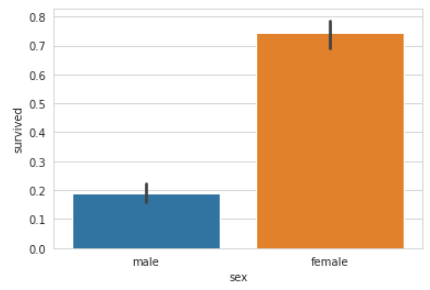Bar Chart Graph Example

Count Plot

`>>> sns.countplot(x="deck", data=titanic)`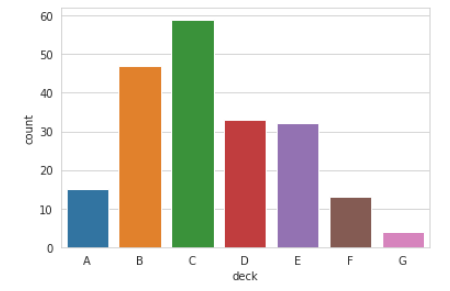Count Plot Graph Example

Point Plot

`>>> sns.pointplot(x="class", y="survived", hue="sex", data=titanic)`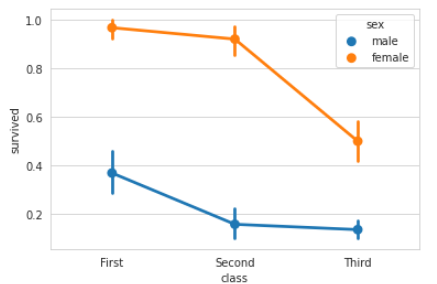Point Plot Graph Example

Boxplot

`>>> sns.boxplot(x="alive", y="age", hue="adult_male", data=titanic)`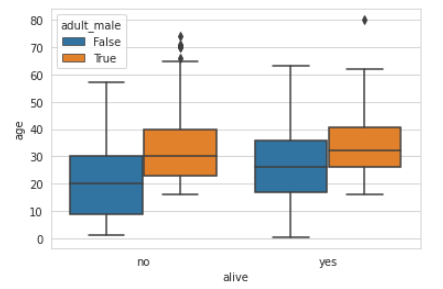Boxplot Graph Example

Violin plot

`>>> sns.violinplot(x="age", y="sex", hue="survived", data=titanic)`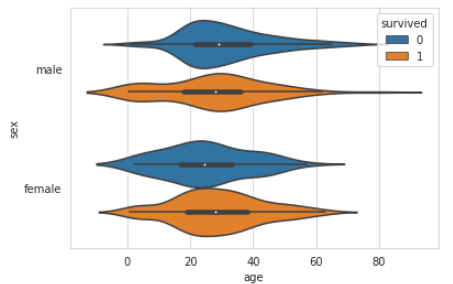Violin plot Graph Example

### Plotting: Regression

`>>> sns.regplot(x="sepal_width", y="sepal_length", data=iris)`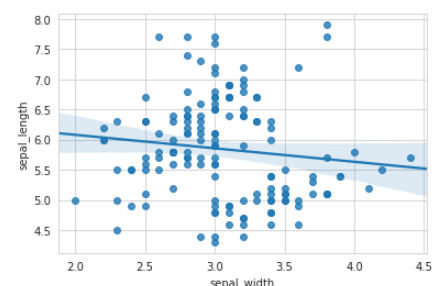Regression Graph Example

### Plotting: Distribution & Matrix

Distribution Plot

`>>> sns.distplot(iris.petal_length)`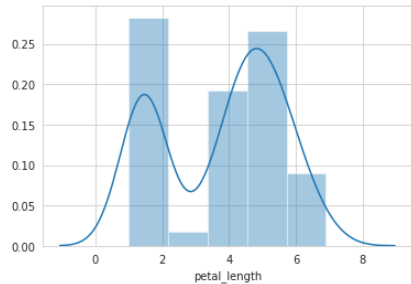Distribution Plot Graph Example

Heatmap

`>>> sns.heatmap(uniform_data, vmin=0, vmax=1)`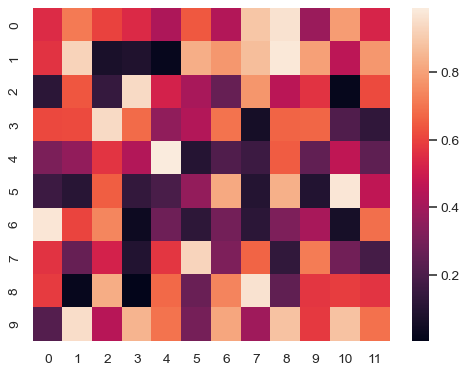Heatmap Graph Example

### Plot Customization

Set axes labels

`>>> g.set_ylabels("Survived")>>> g.set_xlabels("Sex")>>> g.set_axis_labels("Survived", "Sex")`

Set tick labels

`>>> g.set(xlim=(0,5))>>> g.set(ylim=(0,5))`
`>>> g.set(xticks=[0,2.5,5])>>> g.set(yticks=[0,2.5,5)`

### Show and Save Plot

Show Plot

`>>> plt.show()>>> plt.show(g)`

Save Plot

`>>> plt.savefig("foo.png")`

Save Plot: Transparent Figure

`>>> plt.savefig("foo.png", transparent=True)`

### Bonus: Close and Clear

Clear Axis

`>>> plt.cla()`

Clear Figure

`>>> plt.clf()`

Clear Window

`>>> plt.close()`

### Conclusion

I hope you learn something from this cheat sheet. As always, any comments or feedback are highly appreciated!Seaborn Ultimate Cheat Sheet (2021) was originally published in Level Up Coding on Medium, where people are continuing the conversation by highlighting and responding to this story.